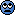Hmm, someone got something backwards somewhere! We want to make the inequalities point the right way: we want

$\mathcal{X}(x,x) \le 0$

so that \$$\mathcal{X}(x,x) = 0\$$ and

$\mathcal{X}(x, z) \le \mathcal{X}(x, y) + \mathcal{X}(y, z)$

Normally it's me who gets things backward, but I thought I just copied the definitions from Fong and Spivak. I'll straighten it out somehow...